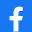# SAP (TFIN52) Financial Accounting II (Lesson :1)

 COMMENTS STADISTICS RECORDS
TAKE THE TEST
 Title of test: SAP (TFIN52) Financial Accounting II (Lesson :1) Description: Organizational Structure Author: Sameer Nirmal(Other tests from this author)Creation Date: 06/08/2012 Category: Competitive Exam Number of questions: 36
 Share the Test:TAKE THE TEST
 Last comments
nomikhanxyz ( uploaded 8 years )
 please correct two answers of the following questions chart of dep always be country specific and dep area under chart of account thanks Answer
Content:
 ________ is denotes the specific logical system you are working on. Client Company Code Chart of Depreciation.________ is an independent accounting Unit. Client Company Code.Business area is to be regarded as a financially separate unit for which an external balance sheet and profit and loss statement are created at this level. True False.Chart of depreciation must be country-specific. True False.Depreciation area come under chart of account. True False.One can not define own depreciation area for a chart of depreciation. True False.Each company code uses one (operative) chart of accounts and one chart of depreciation. True False.All or several company codes can work with the same chart of accounts and the same chart of depreciation. True False.The depreciation areas in a chart of depreciation are defined with a two-digit alphanumeric key. True False.Depreciation area 01 is always what is known as the leading depreciation area. True False.Basic Cost Accounting objects to an asset : Cost center Internal order Activity Type.It is not possible to assign an asset to two cost centers. True False.Asset classes consist of a : Master data section Depreciation area section.Asset classes are created at client level and assigned to at least one chart of depreciation. True False.You can suppress individual depreciation areas per asset class. True False.An asset class can not linked to multiple charts of depreciation. True False.The asset class is the main criterion for classifying assets. True False.Each asset is assigned to only one asset class. True False.You can specify certain control parameters and default values for depreciation calculation and other master data in ______ Chart of depreciation Depreciation key Asset class.There is also at least one special asset class each for assets under construction and low value assets. True False.Depreciation is always posted on a real basis. True False.Depreciation areas post values to the general ledger. True False.Chart of Depreciation defined at the____________ Company Code level Client Level Chart of Account.Chart of depreciation contains pre-defined depreciation areas which represent a specific type of valuation e.g. book depreciation, tax depreciation etc. True False.Different valuation approaches for each asset are mapped in the system by means of depreciation areas. True False.After R/3 enterprise solutions, it is possible to assign additional applications to the asset : Cost Centre and a statistical order Real estate object WBS element A cost centre and a statistical WBS element Maintenance order PSM.Asset class definition applies to all company codes in a chart of accounts. True False.User defined attributes can not overwritten. True False.You can not create intangible assets and leased assets. True False.Depreciation area 01 posts asset values to the general ledger in real time. True False.You can define any number of financial statement versions per chart of accounts in FI. True False.Asset accounting also called Subledgers. True False.Chart of depreciation assigned to ________ Client Compay Code.Depreciation Key combine depreciation area and asset master. True False.Asset master linked to G/L account via A/c determination key. True False.Depreciation key define Terms of Depreciation. True False.
 Report abuse Terms of use
HOME
CREATE TEST
COMMENTS
STADISTICS
RECORDS
Author's Tests